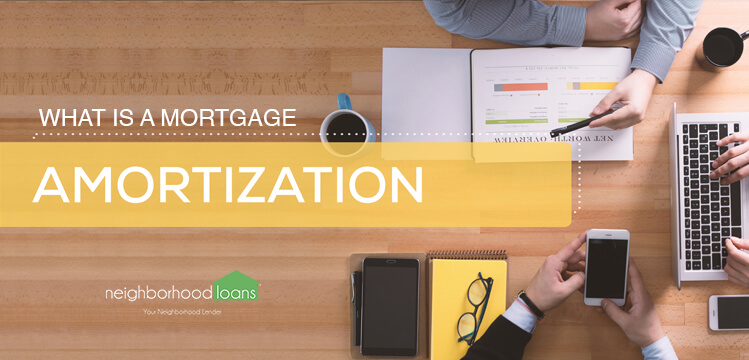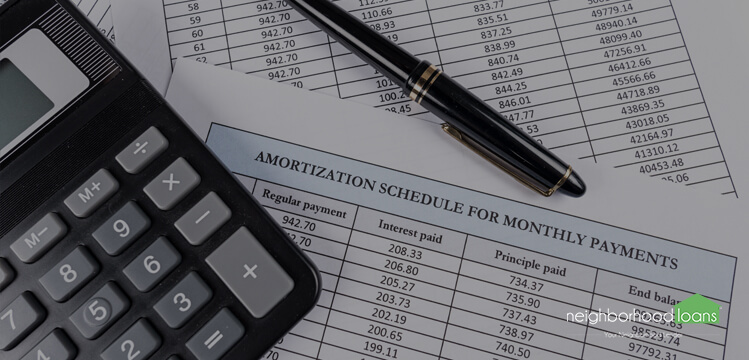As a first-time, repeat, or vacation home buyer, you want to choose the mortgage loan that works best for your needs.

So, how do you choose a loan that works for you?

This ultimately depends on your situation, personally and financially.

Before you start touring homes, it is important to figure out how much you can afford. (If you are wondering how much house you can afford, check out this article, here).

Now, how can you do that?

Well, there’s a way to financially calculate how much you will spend over the loan’s term. This process is known as mortgage amortization.

To understand how this process can be broken down, continue reading.

## What is a mortgage amortization? How does it work?

The percentage of interest and principal in each payment is determined by creating an amortization schedule.

The amortization schedule indicates the exact amount put towards principal and interest with every payment.

Amortization schedules run in chronological order. Meaning, first payments are assumed to take place one full payment period after the loan was taken out, not the following day the loan was processed.

The last payment completely pays off the remainder of the loan. Often, the last payment is a different amount compared to earlier deductions.

Amortization schedules also keep records of the interest paid to date, the principal paid to date, and the remaining principal balance on each payment.

## What is an amortization schedule?

The interest is calculated based on the most recent ending balance of the loan. The interest portion of the loan payment decreases as payments are made.

This is because any payment above the interest amount contributes to reducing the principal, and this reduces the balance in which interest is calculated.

As the interest portion of an amortization loan decreases, the principal portion of the payment increases.

## How are the principal and interest determined?The interest is calculated based on the most recent ending balance of the loan. The interest portion of the loan payment decreases as payments are made.

This is because any payment above the interest amount contributes to reducing the principal, and this reduces the balance in which interest is calculated.

As the interest portion of an amortization loan decreases, the principal portion of the payment increases.

## How is everything calculated?

The current balance of the loan is multiplied by the interest rate for the current period to find the amount of interest due for the period.

Annual interest rates may be divided by 12 to find a monthly rate.

The interest for the period represents the portion of the payment attributable to interest. Therefore, subtracting the interest for the period from the total monthly payment results in the amount of principal paid in the period.

The amount of principal paid in the period is applied to the outstanding balance of the loan. The current balance of the loan minus the amount of the principal paid in the period results in the new outstanding balance of the loan. This new outstanding balance is used to calculate the interest for the next period.

If you’re wondering how you can save some extra money when buying a home, check out this article, here.

## What is an amortization chart?

An amortization chart is a visual explanation of each loan payment, typically for a mortgage loan.

It shows the amount of principal and interest of each payment until the loan is paid off at the end of its term.

The chart shows the total amount of each payment, the due date of each payment, and the amount of principal and interest for each monthly installment.

The terms ‘amortization chart’ and ‘amortization table’ are frequently interchanged, but both define and refer to the same thing.

## What is an amortization calculator?

An amortization calculator, also known as a loan schedule calculator, is used to estimate monthly loan repayments.

Each installment is different due to the amount of interest paid, outstanding balance, and paid principal. There is where an amortization calculator will be useful.

Knowing what each monthly payment will be ahead of time will help you prepare a budget going forward.

For mortgage loan purposes, amortization calculators will need the following information from you: current loan amount, the term (in years), interest rate, and the state you live in.

Amortization schedules fluctuate based on the state you live in, if you’re interested in seeing which state offers the best rates, you can do research online.

## Who should use an amortization calculator?Anyone can use an amortization calculator.

Whether you are a first-time homebuyer, a potential homeowner, or even need a loan for a personal reason, amortization calculators are extremely resourceful.

Amortization calculators can simplify your budget planning by calculating how much you still owe and determine the estimated amount of your regular payments.

If you are curious as to how much you owe or want to budget accordingly, amortization calculators can be a helpful financial tool.

## What do these data entry fields mean?

The data entry fields are straightforward, but here is a little more in-depth explanation of some terms that come up often:

Loan amount: refers to the amount of money you currently owe or the amount you want to borrow.

Interest rate: the proportion of a loan that is charged as interest to the borrower, typically expressed as an annual percentage of the loan outstanding.

Loan Term: This is the time frame you will be making monthly payments to the lender. Once you’ve reached the end of your term, the loan should be paid in full.

## What do these result fields mean?

The ending result can be confusing to some. So, here are the result fields broken down:

Period: This shows the ordinal of payments in the amortization schedule. The total amount of periods can be calculated if you multiply the number of annual periods by the length of years. For example, if you are paid bi-weekly (26 paydays) over 5 years, the loan has 130 periods (26 paydays x 5 years = 130 periods).

Date: This shows the exact date of when the payment is due. Your lender will expect your payments on this date or shortly after.

Interest paid: The amount of your monthly payment that is applied towards interest. As the loan matures, the amount of interest paid decreases.

Principal Paid: shows the specific monetary amount put towards the outstanding balance. As the loan matures, the amount put towards the outstanding principal balance gets larger.

Remaining Balance: also known as outstanding principal balance, is the remaining amount of the loan you owe to your lender. Simply, it is the amount you have not paid yet. This amount can be calculated if your total principal is subtracted from the original loan amount.

### of refinancing?